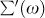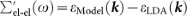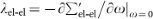## Introduction

The many-body effects, the coupling between electrons and various excitations, are contained in the electron self-energy, which is accessible via the spectral function A(k, ω) measured by angle-resolved photoemission spectroscopy (ARPES) aswhere ε0(k) is the non-interacting (bare) energy-band dispersion and the real and imaginary parts of the self-energy,andaccounts for the energy renormalization and the lifetime broadening, respectively1. Assuming that the k-dependence of the self-energy is negligible,, one can extractandby analyzing the momentum distribution curves (MDCs) at constant energy ω;and, where v0 is the group velocity of the bare band ([planck]−1∂ε0(k)/∂k) and Δk is the MDC half width1. However, the derivation of the self-energy depends on the unfounded assumption of a bare band, which cannot be directly observed. To overcome this difficulty, the self-consistency of the self-energy via Kramers-Kronig relation is the most widely used criteria to extract the self-energy2,5,6,7,8. Moreover, iterative fitting algorithms for the determination of the bare band have also been developed based on the maximum entropy method9,10,11 or the Kramers-Kronig relation12,13,14. In contrast, many previous ARPES experiments have regarded the bare band as a renormalized band due to the electron-electron coupling, which has enabled evaluation of the ‘effective’ bosonic self-energy and coupling strength2,3,4,5,6,7,8. However, the term ‘effective’ is often abbreviated or overlooked in many cases, although it has been proposed that the effective electron-boson coupling strength is smaller than the true coupling strength because of the electron-electron coupling15,16. There is a need, therefore, to reconsider and/or refine the most commonly used method for extracting the bosonic self-energy from ARPES data.

## Results

We assume two main scattering channels, the electron-boson and electron-electron couplings. The energy-scales associated with these two are quite different: the electron-boson coupling dominates at low energy-scales (at most ~100 meV), whereas the electron-electron coupling over a wide energy-scale (eV-order). The two are thus nearly independent in the scattering process and hence the Matthiessen's rule holds17. Then, the total self-energy and coupling strength can be written as the summation of the contributions from these couplings;and λtot = λel-boson + λel-el.

Figure 1 schematically illustrates the energy-band renormalizations: the bare dispersion ε0(k) (black) is renormalized due to the electron-electron coupling in the wide energy range resulting in εel-el(k) (red) and further renormalized by the electron-boson coupling near the Fermi level resulting in εtot(k) (blue). Note that εel-el(k) corresponds to the ‘bare’ dispersion that has been extensively used so far. Here, we focus on the bosonic real-part of the self-energy, which can be defined at k = k0 aswhere we used relationsand. Also, the group velocities of ε0(k) and εel-el(k) are given by v0 = (ε1 − ε0)/(k1k0) and, respectively, yieldingwithin the effective mass approximation. Now we can rewrite equation (2) asHere, we introduced, which is equivalent to the ‘effective’ bosonic real part of the self-energy, namely, the energy shift due to the electron-boson coupling measured from, the renormalized energy arising from the electron-electron coupling. Consequently, the magnitude of the ‘effective’ bosonic real part of the self-energy is reduced by a factor of (1 + λel-el). Therefore, for the proper evaluation of the ‘true’ electron-boson coupling, especially for strongly correlated electron systems, it is indispensable to account for the electron-electron coupling.

Similarly, the coupling strength can be deduced by differentiating equation (3) aswhereand.

We emphasize the point that the problem with the conventional procedure is indeed simple and can be explicitly summarized by the following:Comparing with equation (1), the energy reference point clearly differs for the electron-electron coupling, despite the summation of the real part of the self-energy having to be taken at the same energy ε*. As a result, the magnitude of the real part of the self-energy due to the electron-electron coupling is overestimated, producing subsequently an underestimated electron-boson coupling.

Similar arguments, described in Supplementary Information, also hold for the imaginary part of the self-energy. The ‘true’ and ‘effective’ bosonic real and imaginary parts of the self-energy only differs by factor (1 + λel-el), indicating that not only the ‘true’ but also the ‘effective’ bosonic self-energy should satisfy the Kramers-Kronig relation. This means that the self-consistency of the self-energy does not solely guarantee the validity of the bare band as well as the magnitude of the bosonic self-energy.

Based on the above methodology, we have examined the ‘true’ coupling strength of the electron-boson coupling in the unconventional ruthenate superconductor Sr2RuO4. The Fermi surface (FS) and low-lying electronic structure of Sr2RuO4 has been established by de Haas-van Alphen (dHvA), ARPES and local-density approximation (LDA) band-structure calculations18,19. There are three FSs in Sr2RuO4 (see Fig. 2a): one hole-like α FS around the X point and two electron FSs (β and γ) around the Г point. The effective mass enhancements were also determined by dHvA experiments as m*/mLDA (α, β, γ) = (3.0, 3.5, 5.5)18, where m* and mLDA is the effective mass and the LDA band mass, respectively. However, this poses a long-standing question as to why the largest mass enhancement emerges in the γ FS formed by the widest dxy band (Wxy ~ 3 eV) and not in the α and β FSs formed by the respective narrow dyz and dzx bands (Wyz,zx ~ 1 eV). We have therefore performed high-resolution ARPES measurements (see Methods) along the ГM line, where the γ band can be almost solely observed, as was found in our previous polarization-dependent ARPES study20.

Figure 2b shows the γ band dispersion derived from ARPES (blue circles), model calculations (red) and LDA calculations (black). The model calculations include band renormalization effects due to the electron-electron coupling as well as the spin-orbit coupling, both of which were empirically evaluated based on ARPES data presented elsewhere20,21,22 (see also Supplementary Information). The γ-quasiparticle parameter values, determined from these dispersions, are listed in Table 1; the values obtained from ARPES are found to be close to dHvA results18. The slight difference between the two results can be reasonably explained by instrument resolution, which distort the quasiparticle dispersion near the Fermi level (see Supplementary Information). With the available renormalized mass listed in Table 1, we can now disentangle the electron-boson and electron-electron coupling strengths:,and thus λel-boson = λtot − λel-el = 1.2. The ‘effective’ bosonic coupling strength can be also calculated as. Note that one can confirm that equation (4) actually holds for these values.

The real part of the self-energies, given in Fig. 2c, can be extracted by subtracting two dispersions:,and, which are marked with black open circles, a black solid line and blue filled circles, respectively. We found that the summation ofand(blue open circles) is completely different from the, indicating that. By contrast, the summation ofand(red open circles) precisely coincides with, thus satisfying equation (1). Here,(red filled circles) was derived frommultiplied by (1 + λel-el), where. The coupling strengths, obtained from the derivative of the real part of the self-energy, are listed in Table 2; all are in good agreement with those obtained from the effective mass enhancement. Therefore, the present ARPES results clearly demonstrate the validity of our self-energy analysis.

## Discussion

Contrary to the rather small ‘effective’ bosonic coupling strength, the ‘true’ bosonic coupling strength is found to be as strong as λel-boson = 1.1–1.2 in Sr2RuO4, which is a clear indication of strong coupling (e.g., see Ref. 23). Note that λel-boson probably becomes ~2.0 if we consider instrument resolution (see Supplementary information). Such strong bosonic coupling can be expected to originate from the multiple bosonic couplings20 and its key ingredient could have particular relevance to the pairing mechanism, although at present we are unable to resolve this given the limited resolution as well as statistics.

Our results clearly outline the problem with the conventional self-energy analysis, which often leads to an underestimation of the bosonic coupling strength by a factor of (1 + λel-el). The new insight we have presented here points toward a need for a critical revision of the bosonic coupling strength, that could probably rationalize the strong electron-boson coupling in strongly correlated superconductors15,24.

## Methods

High-quality Sr2RuO4 single crystals were grown by the floating zone method with the self-flux technique25. Polarization-dependent ARPES measurements were performed at the linear undulator beamline (BL-1) of the Hiroshima Synchrotron Radiation Center using a high-resolution, hemispherical electron analyzer (R4000, VG-SCIENTA). All measurements were performed after cleaving the samples in situ under ultra-high-vacuum conditions (~2 × 10−11 Torr) at temperatures lower than 10 K. To eliminate the surface states of Sr2RuO4, the sample surface was aged and then, ARPES data were collected at the photon energy of 42-eV with the s-polarization geometry26. The polarization geometry, excitation energy and location of the Brillouin zone were carefully determined from the ARPES data as described elsewhere20,21. The energy and angular resolutions were set to better than 10 meV and 0.08°, respectively.